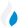•Trade Pi
•Trade
•Exchange
•Trade Pi
•Trade
•Exchange
\$1.08T
Total marketcap
\$61.34B
Total volume
41.07%
BTC dominance
•Trade Pi
•Trade
•Exchange

# MAXI to HDRN Exchange Rate - 1 Maximus DAO in Hedron

21,033.947928379
Vol [24h]
\$168.08

## maxi to hdrn converter

Exchange Pair Price 24h volume
Uniswap (v3) MAXI/HDRN \$0.032 \$168.08

## MAXI/HDRN Exchange Rate Overview

Name Ticker Price % 24h 24h high 24h low 24h volume
Maximus DAO maxi \$0.03613 2.3595% \$0.0364 \$0.03437 \$13.74K
Hedron hdrn \$0.000001729 8.171% \$0.00000174 \$0.000001579 \$1.49M

Selling 1 MAXI you get 21,033.947928379 Hedron hdrn.

Maximus DAO Aug 11, 2022 had the highest price, at that time trading at its all-time high of \$0.1261.

174 days have passed since then, and now the price is 28.66% of the maximum.

Based on the table data, the MAXI vs HDRN exchange volume is \$168.08.

Using the calculator/converter on this page, you can make the necessary calculations with a pair of Maximus DAO/Hedron.

## Q&A

### What is the current MAXI to HDRN exchange rate?

Right now, the MAXI/HDRN exchange rate is 21,033.947928379.

### What has been the Maximus DAO to Hedron trading volume in the last 24 hours?

Relying on the table data, the Maximus DAO to Hedron exchange volume is \$168.08.

### How can I calculate the amount of HDRN? / How do I convert my Maximus DAO to Hedron?

You can calculate/convert HDRN from Maximus DAO to Hedron converter. Also, you can select other currencies from the drop-down list.

## MAXI to HDRN Сonversion Table

MAXI HDRN
20 MAXI = 420,678.9 HDRN
30 MAXI = 631,018.4 HDRN
60 MAXI = 1,262,036.8 HDRN
90 MAXI = 1,893,055.3 HDRN
200 MAXI = 4,206,789.5 HDRN
300 MAXI = 6,310,184.3 HDRN
500 MAXI = 10,516,973.9 HDRN
2000 MAXI = 42,067,895.8 HDRN
3000 MAXI = 63,101,843.7 HDRN
30000 MAXI = 631,018,437.8 HDRN
300000 MAXI = 6,310,184,378.5 HDRN
3000000 MAXI = 63,101,843,785.1 HDRN
30000000 MAXI = 631,018,437,851.3 HDRN